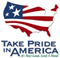# Use of RORA for Complex Ground-Water Flow Conditions

## Abstract

The RORA computer program for estimating recharge is based on a condition in which ground water flows perpendicular to the nearest stream that receives ground-water discharge. The method, therefore, does not explicitly account for the ground-water-flow component that is parallel to the stream. Hypothetical finite-difference simulations are used to demonstrate effects of complex flow conditions that consist of two components: one that is perpendicular to the stream and one that is parallel to the stream. Results of the simulations indicate that the RORA program can be used if certain constraints are applied in the estimation of the recession index, an input variable to the program. These constraints apply to a mathematical formulation based on aquifer properties, recession of ground-water levels, and recession of streamflow.

## Introduction

The RORA computer program estimates recharge to the water table on the basis of daily streamflow (Rutledge, 1998; 2000). The program is intended for analysis of a ground-water-flow system driven by diffuse areal recharge to the water table and by ground-water discharge to a gaining stream. When the program is used, regulation and diversion of streamflow should be negligible.

The formulations that RORA uses are derived from a cross-sectional-flow model that calculates ground-water discharge per unit of stream length on the basis of designated transmissivity, storage coefficient, distance from the stream to the hydrologic divide, and increase in head caused by recharge to the ground-water system (Rorabaugh, 1964, p. 433). The program is based on simple geometry, as illustrated in figure 1A, which represents a segment of a basin. The bold line on the left border represents the stream, and the right border represents the hydrologic divide. Because the stream extends along the length of the model, and because its altitude is uniform, the direction of ground-water flow is one-dimensional and perpendicular to the stream. A finite-difference-flow model, MODFLOW-96, then is used to generate the hydrographs of ground-water level and ground-water discharge to the stream (fig. 1B, details about this simulation are given in “Finite-Difference Simulation Design”).

Before applying RORA, the user must designate the recession index (K). The input variable K is a measure of the time required for ground-water discharge to recede by one log cycle when the recession becomes linear (or nearly linear) on the semilog hydrograph. In the ideal model (fig. 1), K can be determined from the following equation, which is derived from Rorabaugh and Simons (1966, p. 12) as:

 0.933a2S K = T
(1)

where a is the distance from the stream to the hydrologic divide (same as arrows in fig. 1); S is storage coefficient; and T is transmissivity. Hydrographs of ground-water level and ground-water discharge (fig. 1) show linearity of recession on the semilog plot. When RORA is used in this ideal simulation, the determination of K (and subsequent application of RORA) is straightforward because:

1. the value of “a” is not ambiguous;
2. the recession of ground-water level (when expressed as vertical distance above the altitude of a known outflow boundary) is clearly definable and is equal to K;
3. streamflow recession is equal to K, some time after recharge;
4. there are no departures from linearity in the semilog plot of recession.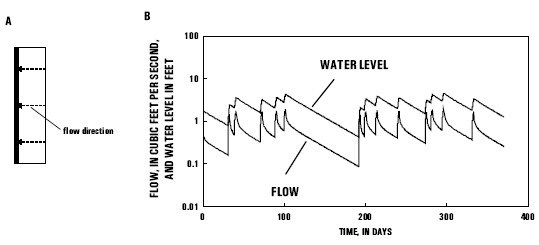Figure 1. (A) Finite-difference-flow model (plan view) and (B) simulated flow to drains and simulated ground-water level. (Water levels are shown for the finite-difference cell in the middle of the model.)

When a component of ground-water flow is parallel to the stream, in the “downvalley” direction toward the next stream in the network, that component will cause departures from the ideal conditions described in items 1-4. This report demonstrates the use of the RORA program for these complex flow conditions. Finite-difference simulations are used to evaluate flow conditions. In one simulation, flow is perpendicular to the stream (fig. 1). In other simulations, there is an additional flow component that is parallel to the stream. The latter simulations include a variety of outflow-boundary slopes.

## Finite-Difference Simulation Design

Each of the three finite-difference simulations (fig. 2) includes drain cells that represent streams. In simulation A, drain cells are configured so that all flow will be one-dimensional, in the “west” direction, perpendicular to the stream (same as fig. 1). The altitude of all drain cells is zero in simulation A. In simulations B and C, drain cells are configured so that a flow component will be parallel to the stream. This configuration includes an absence of drain cells in the northern part of the model and additional drain cells along the southern boundary. The altitude of the additional drains along that boundary is zero. The altitude of drains along the western boundary increases from zero at the southern end to some higher altitude at the northern boundary. Slopes of drain lines are uniform and are expressed as SB and SC, in feet per 100 feet (ft/100 ft). Included among simulations are two variations on the flow condition illustrated in figure 2. In the first variation, SB = 0.05 and SC = 0.25 ft/100 ft; and in the second variation, SB = 0.4 and SC = 2.0 ft/100 ft. (These slopes correspond to about 3, 13, 21, and 106 feet per mile.) The conductance of all drain cells is uniform (0.1 feet squared per second).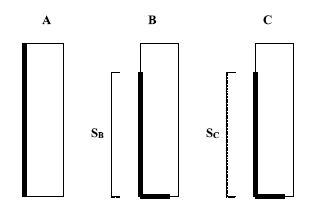Figure 2. The three finite-difference simulations used to evaluate flow conditions (plan view): (A) simulation with flow perpendicular to the stream, (B and C) simulations that include a flow component that is parallel to the stream. (Each simulation measures 8,000 ft in the north-south direction and 2,000 ft in the east-west direction. Aquifer properties are uniform. Bold lines indicate drains. The altitude of all drains is zero, except for the segments SB and SC, which are sloping drains. The altitude of these segments is zero at the southern boundary.)

The program MODFLOW-96 (Harbaugh and McDonald, 1996) is used to simulate flow. Each model measures 8,000 ft in the north-south direction and 2,000 ft in the east-west direction. All finite-difference cells are squares measuring 100 ft on the side. In each simulation, storage coefficient and transmissivity are uniform. Drain segments are intended to represent streams that receive ground-water discharge most of the time, with the exception of extreme drought. The initial hydraulic-head distribution in each simulation is calculated on the basis of a preliminary steady-state simulation in which ground-water recharges at a constant rate of 10 in/yr (inches per year). Subsequently, all simulations include 13 recharge events, each lasting 2 days, and each uniformly inducing 1 inch of recharge.

## Simulation Results

Simulation results are shown for different transmissivities and storage coefficients (figs. 3 and 4). In each group of three simulations, recession characteristics of simulations B and C are similar to recession characteristics of simulation A. Characteristics in simulation A must be dominated by the flow component that is perpendicular to the length of the stream; therefore, it appears that component also is dominating recession characteristics in simulations B and C. Consequently, if equation 1 is used to determine the recession index before the RORA program is applied, the value of “a” might be measured in the “east-west” direction in each simulation. To test this hypothesis, the RORA program is used to calculate recharge from each model-generated data set of ground-water discharge. Before application of RORA, the recession index is calculated from equation 1, designating a = 2,000 ft. The following tabulation shows the recession index and recharge estimated using RORA:

 Figure 3 Simulation Recession index (days per log cycle) Recharge (inches) A 93 11.6 B 93 11.3 C 93 11.2 Figure 4 A 373 11.7 B 373 11.5 C 373 11.5

The RORA program does not detect the last recharge event, but this is not ordinarily important because the program is intended for analysis of long periods (years) of streamflow record. The recharge simulated by MODFLOW, for which RORA is giving estimates, is equal to 12 inches.

All simulations described include an initial head distribution that was calculated by using a preliminary steady-state simulation, in which recharge is 10 in/yr. To test effects of initial conditions, all simulations were run again, but with a steady-state recharge of 20 in/yr (not illustrated). The RORA program was used to estimate recharge for each of the six simulated flow records. Each result varied from its corresponding result previously presented, by 2 percent or less.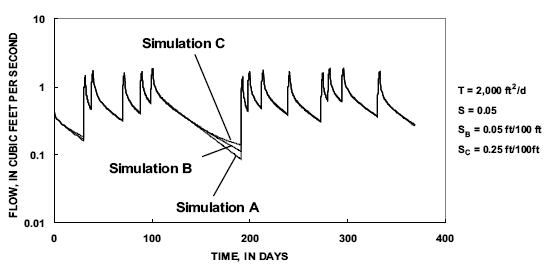Figure 3. Ground-water discharge in the three simulations, with hydraulic diffusivity = 40,000 feet squared per day (ft2/d). (Simulation A is identical to the simulation in figure 1. Variables are transmissivity (T), Storage coefficient (S), and slopes of drain lines in simulations B and C (SB and SC, respectively).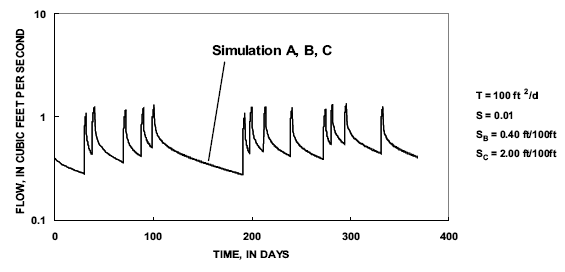Figure 4. Ground-water discharge in the three simulations, with hydraulic diffusivity = 10,000 feet squared per day (ft2/d). (Variables are transmissivity (T), Storage coefficient (S), and slopes of drain lines in simulations B and C (SB and SC, respectively).)

Model-generated hydraulic head for a simulation that includes complex flow will indicate considerable variation in the direction of flow, depending on the time elapsed since the last recharge event (fig. 5). In some hydrologic studies, the length of a ground-water-flow path might be used to designate “a” in equation 1. In this simulation, the length of a flow path might vary from 2,000 to 8,000 ft, depending on the time that is represented by the water-table map, and the location of the specific flow path. The RORA program was used to estimate recharge for all simulations illustrated in figures 3 and 4, substituting 4,000 and 8,000 ft for the variable “a” in equation 1. When these longer flow paths are considered to represent “a,” the estimates of recharge calculated by RORA demonstrated greater error than the errors when “a” was designated as 2,000 ft. Errors were particularly large when 8,000 ft was substituted. In this case, the estimate of recharge calculated by RORA exceeds the MODFLOW-designated recharge by a factor from 1.7 to 2.9. As indicated above, it appears that if the recession index is calculated from equation 1, then “a” should be measured in the direction that is perpendicular to the stream, or transverse from the stream to the hydrologic divide, not from flow-path analysis.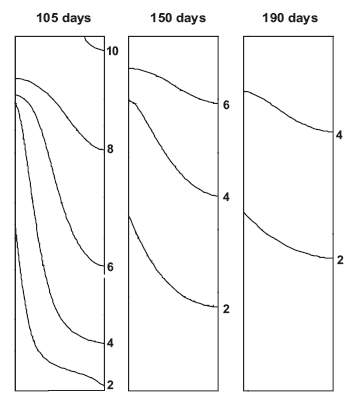Figure 5. Ground-water levels in the finite-difference simulation illustrated in figure 2B, at time = 105, 150, and 190 days (plan view). (Numbered contours represent equal ground-water level, in feet. The hydrograph of ground-water discharge is figure 3B.)

For the ideal condition in which all ground-water flows perpendicular to the stream, the recession of the ground-water level will equal the recession index. Importantly, the variable considered should be defined as the altitude of the water level in the well minus the altitude of the outflow boundary. Because the altitude of the outflow boundary in figure 1 is uniformly equal to zero, subtraction is not necessary. Simulations of complex flow conditions, however, include variation in altitude of the outflow boundary. It could be inferred from such simulations (fig. 5) that the orientation and direction of flow paths will change over time. This change, in turn, would lead to various interpretations of the location of the outflow boundary. Recession rates, expressed in days per log cycle, vary depending on selection of the location of the outflow boundary for a simulation in which the altitude of this boundary changes along its length (fig. 6). It appears that if ground-water-level recession is used to ascertain the recession index, results can vary appreciably.Figure 6. (A) A location at which ground-water level is considered and five drain locations (plan view), and (B) ground-water-level recession expressed as the difference between water level at the point of interest and the altitude of the drain. (The five different drain locations are indicated with numbers on frame A, and the recession curves are labeled with the corresponding number on frame B. The simulation shown is the same as the simulation shown in figures 2B and 3B.)

The most practical method for determining K is measuring recession rates directly from the semilog flow hydrograph. Rutledge (2000, p. 14-18) includes discussion of this method and potential problems. The method, which was used in a regional application of RORA (Rutledge and Mesko, 1996), consists of locating a part of the flow hydrograph that shows continuous recession, finding a segment of recession that begins a sufficient amount of time after recharge so that it will exhibit linearity on the semilog plot, then determining the slope in days per log cycle. To test this method for the hydrographs in figure 3, the flow from day 120 to 130 is considered to represent a linear-recession segment. As an approximation of the graphical method of calculating the recession index, the difference between the logarithm of flow on these 2 days is calculated, then this rate of change is converted to days per log cycle. A method using daily flows at 150 and 160 days was used for the hydrographs in figure 4. The following tabulation shows the recession index, thus determined, and the recharge calculated using RORA.

Simulation Recession index Recharge (days per log cycle) (inches) Figure 3 A 89 11.5 B 94 11.3 C 94 11.2 Figure 4 A 314 11.4 B 319 11.3 C 319 11.3

The estimates for the recession index (K) from the hydrograph in figure 3 almost are equal to the result of equation 1 (the equation gives 93 days/log cycle). The estimates for the recession index from the hydrograph in figure 4, however, vary considerably from the result of the equation (the equation gives 373 days/log cycle). Nonetheless, the estimates of recharge from this method are similar to estimates of recharge presented earlier.

Given the quantitative similarity between hydrographs affected by complex flow conditions and hydrographs not affected by them, recession characteristics under extreme low-flow conditions should not be used when K is determined. This would apply to departures from the linear model of recession, such as exhibited by curves B and C in figure 3, in the time between 170 and 190 days.

As discussed, the ideal condition (fig. 1) exhibits a perfect log-linear relation in the recession of the streamflow hydrograph, after a period since the last recharge event. Complex flow causes slight nonlinearity (figs. 3 and 4). The program user should evaluate variation in recession characteristics for the basin of interest. In many cases, the recharge estimate may not be extremely sensitive to variation in K.

## Conclusions

The RORA computer program for estimating recharge is based on a condition in which ground water flows perpendicular to the nearest stream that receives ground-water discharge. The program does not explicitly account for the component of flow that is parallel to the stream. The program can be used for these flow conditions, if certain constraints are applied in the estimation of the recession index (K), an input variable to the program. These constraints are as follows:

1. If the equation 0.933(a2)S/T is used to determine K, the value of “a” should be measured as the transverse distance from the stream to the hydrologic divide. Estimation of “a” based on flow-path lengths may introduce errors.
2. If K is determined from the recession of ground-water levels, results can vary depending on the user’s selection of the outflow boundary location. The boundary location can be open to interpretation because there may be multiple flow paths.
3. If K is determined from streamflow data, extreme low-flow conditions should not be used.

The most practical method for estimating K is from analysis of the streamflow data set. Program users should be cautious when attempting to evaluate or replace this estimate on the basis of flow paths or ground-water levels.

## References Cited

Harbaugh, A.W., and McDonald, M.G., 1996, User’s documentation for MODFLOW-96, an update to the U.S. Geological Survey modular finite-difference ground-water flow model: U.S. Geological Survey Open-File Report 96-485, 56 p.

Rorabaugh, M.I., 1964, Estimating changes in bank storage and ground-water contribution to streamflow: International Association of Scientific Hydrology, Publication 63, p. 432-441.

Rorabaugh, M.I., and Simons, W.D., 1966, Exploration of methods relating ground water to surface water, Columbia River Basin–second phase: U.S. Geological Survey Open-File Report 66-117, 62 p.

Rutledge, A.T., 1998, Computer programs for describing the recession of ground-water discharge and for estimating mean ground-water recharge and discharge from streamflow data–update: U.S. Geological Survey Water-Resources Investigations Report 98-4148, 43 p. url: https://pubs.water.usgs.gov/wri98-4148

Rutledge, A.T., 2000, Considerations for use of the RORA program to estimate ground-water recharge from streamflow records: U.S. Geological Survey Open-File Report 00-156, 44 p. url: https://pubs.water.usgs.gov/ofr00-156

Rutledge, A.T., and Mesko, T.O., 1996, Estimated hydrologic characteristics of shallow aquifer systems in the Valley and Ridge, the Blue Ridge, and the Piedmont Physiographic Provinces based on analysis of streamflow recession and base flow: U.S. Geological Survey Professional Paper 1422-B, 58 p.

The citation for this report, in USGS format, is as follows:

Rutledge, A.T., 2003, Use of RORA for complex ground-water flow conditions: U.S. Geological Survey Water-Resources Investigations 03-4304, 5 p.

 AccessibilityFOIAPrivacyPolicies and Notices U.S. Department of the Interior, U.S. Geological Survey Persistent URL: Page Contact Information: Contact USGS Last modified: Wednesday, December 07 2016, 01:21:25 PM